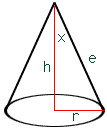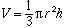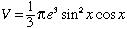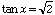I need help with this problem for my high school calculus class. Any help you can give me will be greatly appreciated-I am pretty stumped. "Express the volume of a cone in terms of the slant height 'e' and the semi-vertical angle 'x' and find the value of 'x' for which the volume is a maximum if 'e' is constant." Thank you. Jocelyn Wozney Hi Jocelyn, Below is my diagram, but I am not sure I have the correct angle x.The volume of a cone isFrom the diagram r = e sin(x) and h = e cos(x) and henceTo find the value of x which maximizes the volume, differentiate V with respect to x, set the derivative to zero and solve for x. This leads toCheers, Harley Go to Math Central